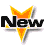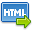Volume No. :   9

Issue No. :  4

Year :  2019

ISSN Print :  2231-5705

ISSN Online :  2231-5713Registration

## Determination of Concentration of Zolmitriptan in Solid Dosage Form by UV Spectroscopic Method

 Address: Shubhangi C. Daswadkar1*, Jyoti D Gaikwad2 1Department of Pharmaceutical Chemistry, Dr. D. Y. Patil College of Pharmacy, Akurdi, Pune – 411044 India. 2Department of Pharmaceutical Quality Assurance Techniques, Dr. D. Y. Patil College of Pharmacy, Akurdi, Pune- 411044 India. *Corresponding Author
DOI No: 10.5958/2231-5713.2019.00040.0

ABSTRACT:
Zolmitriptan (ZMT) is a drug used in the treatment of migraines and cluster headaches.ZMT in bulk and pharmaceutical formulation determined by Zero order, First order derivative, Second order derivative and AUC by using UV-spectroscopic methods. The standard stock solution was prepared of 100 µg/ml for all proposed methods, and working standard solutions were prepared from the stock solution after adequate dilutions (1-10 µg/ml).For zero order derivative, standard solutions were measured at 283nm.For first order derivative spectroscopy the response (dA/d?) of standard solutions was measured at 216 nm. Calibration curve was constructed by plotting response (dA/d?) values against concentrations. Regression equation of linear calibration graph was calculated as (r2=0.994). The response for second order derivative spectroscopy was measured at 237nm,and the regression equation was calculated as (r2=0.992).The AUC-spectroscopic method was based on calculation of area under curve (AUC) for analysis of zolmitriptan in the wavelength range of278-283nm,regression equation of linear calibration graph was calculated as (r2=0.993). All Developed methods were validated as per ICH guidelines. Hence the developed methods are used for routine analysis of solid dosage form containing Zolmitriptan.
KEYWORDS:
Zolmitriptan, UV-spectroscopy, AUC- spectroscopy.
Cite:
Shubhangi C. Daswadkar, Jyoti D Gaikwad. Determination of Concentration of Zolmitriptan in Solid Dosage Form by UV Spectroscopic Method. Asian J. Pharm. Tech. 2019; 9(4):244-248.[View HTML]

Visitor's No. :   219741